###### Performing A/B test in Python example – A case study from Udacity Data Scientist Nano Degree
2022-08-24###### A tutorial on Scikit-Learn Pipeline, ColumnTransformer, and FeatureUnion
2022-08-30Cross-validation is an important concept in machine learning which helps the data scientists in two major ways: it can reduce the size of data and ensures that the artificial intelligence model is robust enough. Cross-validation does that at the cost of resource consumption, so it’s important to understand how it works before you decide to use it.

In this article, we will briefly review the benefits of cross-validation and afterward, I’ll show you detailed applications using a broad variety of methods in the popular python Sklearn library. We will learn:

• What are KFold, ShuffledKfold, and StratifiedKfold and see how they differ
• How to cross-validate your model without KFold using cross_validate and cross_val_score methods
• What are the other split options — RepeatedKFold, LeaveOneOut, and LeavePOut and a use case for GroupKFold
• How important it is to consider target and feature distribution

## Benefit 1: Data size reduction

Normally you split the data into 3 sets.

• Training: used to train the model and optimize the model’s hyperparameters
• Testing: used to check that the optimized model works on unknown data to test that the model generalizes well
• Validation: during optimizing some information about the test set leaks into the model by your choice of the parameters so you perform a final check on completely unknown data

Introducing cross-validation into the process helps you to reduce the need for the validation set because you’re able to train and test on the same data.

In the most common cross-validation approach, you use part of the training set for testing. You do it several times so that each data point appears once in the test set.

## Benefit 2: Robust process

Even though sklearn’s train_test_split method is using a stratified split, which means that the train and test set have the same distribution of the target variable, it’s possible that you accidentally train on a subset that doesn’t reflect the real world.

Imagine that you try to predict whether a person is a male or a female by his or her height and weight. One would assume that taller and heavier people would rather be males; though if you’re very unlucky your train data would only contain dwarf men and tall Amazon women. Thanks to cross-validation you perform multiple train_test split and while one fold can achieve extraordinary good results the other might underperform. Anytime one of the splits shows unusual results it means that there’s an anomaly in your data. If your cross-validation split doesn’t achieve a similar score, you have missed something important about the data.

## Cross-Validation in Python

You can always write your own function to split the data, but scikit-learn already covers 10 methods for splitting the data which allows you to tackle almost any problem.

As a first step let’s create a simple range of numbers from 1,2,3 … 24,25.

```# create the range 1 to 25
rn = range(1,26)
```

Then let’s initiate the sklearn Kfold method without shuffling, which is the simplest option for how to split the data. I’ll create two Kfolds, one splitting data 3-times and the other doing 5 folds.

```from sklearn.model_selection import KFold
kf5 = KFold(n_splits=5, shuffle=False)
kf3 = KFold(n_splits=3, shuffle=False)
```

If I pass my range to the KFold it will return two lists containing indices of the data points which would fall into the train and test set.

```# the Kfold function retunrs the indices of the data. Our range goes from 1-25 so the index is 0-24
for train_index, test_index in kf3.split(rn):
print(train_index, test_index)
```

KFold returns indices, not the real data points. Since KFold returns the index, if you want to see the real data we must use `np.take` in NumPy array or `.iloc` in pandas.

```# to get the values from our data, we use np.take() to access a value at particular index
for train_index, test_index in kf3.split(rn):
print(np.take(rn,train_index), np.take(rn,test_index))
```

## How does KFold split the data?

To better understand how the KFold method divides the data, let’s display it on a chart. Because we have used `shuffled=False` the first data point belongs to the test set in the first fold, the next one as well. The test and train data points are nicely arranged.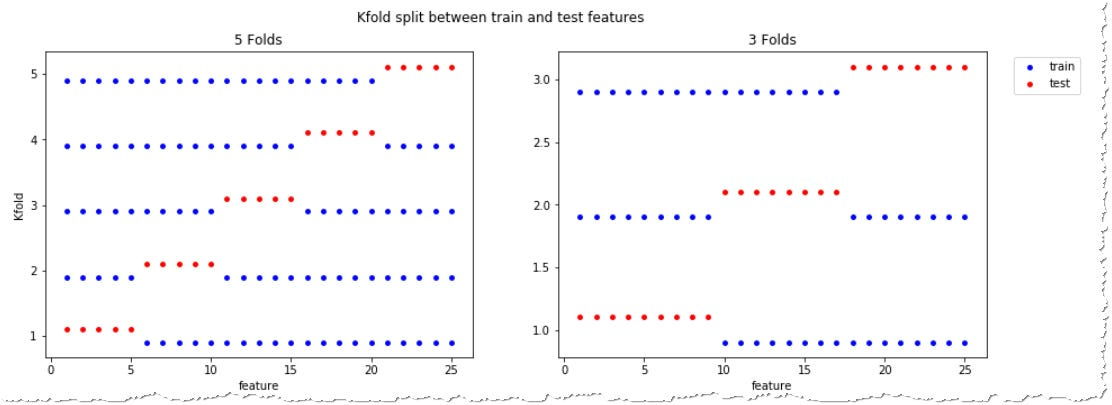Unshuffled KFold split having 5 and 3 folds, charts code on Github

It’s important to say that the number of folds influences the size of your test set. 3 folds tests on 33% of the data while 5 folds on 1/5 which equals 20% of the data. Each data point appears once in the test set and k-times in the train set.

## Shuffled KFold

Your data might follow a specific order and it might be risky to select the data in order of appearance. That can be solved by setting KFold’s shuffle parameter to `True`. In that case, KFold will randomly pick the data points which would become part of the train and test set. Or to be precise not completely random, random_state influences which points appear in each set and the same `random_state` always results in the same split.

## KFold using the real dataset

Working on the real problem you will rarely have a small array as an input so let’s have a look at the real example using a well-known Iris dataset. Iris dataset contain 150 measurements of petal and sepal sizes of 3 varieties of the iris flower —50 Iris setosas, 50 Iris virginicas and 50 Iris versicolors

## When KFold cross-validation runs into a problem

In the GitHub notebook, we run a test using only a single fold which achieves 95% accuracy on the training set and 100% on the test set. My surprise was when the 3-fold split resulted in exactly 0% accuracy. You read it well, my model did not pick a single flower correctly.

```i = 1
for train_index, test_index in kf3.split(iris_df):
X_train = iris_df.iloc[train_index].loc[:, features]
X_test = iris_df.iloc[test_index][features]
y_train = iris_df.iloc[train_index].loc[:,'target']
y_test = iris_df.loc[test_index]['target']

#Train the model
model.fit(X_train, y_train) #Training the model
print(f"Accuracy for the fold no. {i} on the test set: {accuracy_score(y_test, model.predict(X_test))}")
i += 1
```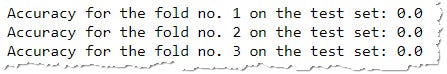The accuracy of the machine learning model was 0% on each fold. Why?

Do you remember that unshuffled KFold picks the data in order? Our set contains 150 observations, the first 50 belongs to one specie, 51–100 to the other, and the remaining to the third one. Our 3-fold model was very unlucky to always pick the measurement of two of the Irises while the test set contained only the flowers that the model has never seen.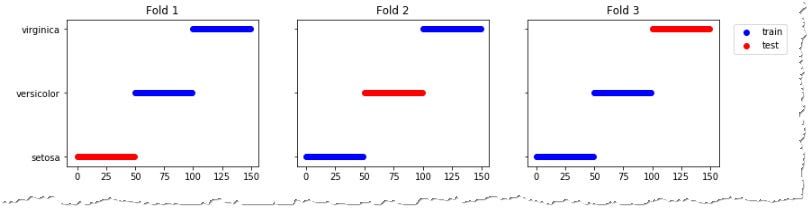The first fold failed to recognize Iris Setosa in the test set which it never saw because it was trained on Virginia and Versicolor.

## Cross-Validation example using K-fold method

```#Importing required libraries
import pandas as pd
from sklearn.model_selection import KFold
from sklearn.linear_model import LogisticRegression
from sklearn.metrics import accuracy_score

df = data.frame
X = df.iloc[:,:-1]
y = df.iloc[:,-1]

#Implementing cross validation

k = 5
kf = KFold(n_splits=k, random_state=None)
model = LogisticRegression(solver= 'liblinear')

acc_score = []

for train_index , test_index in kf.split(X):
X_train , X_test = X.iloc[train_index,:],X.iloc[test_index,:]
y_train , y_test = y[train_index] , y[test_index]

model.fit(X_train,y_train)
pred_values = model.predict(X_test)

acc = accuracy_score(pred_values , y_test)
acc_score.append(acc)

avg_acc_score = sum(acc_score)/k

print('accuracy of each fold - {}'.format(acc_score))
print('Avg accuracy : {}'.format(avg_acc_score))

# accuracy of each fold - [0.9122807017543859, 0.9473684210526315, 0.9736842105263158, 0.9736842105263158, 0.9557522123893806]
# Avg accuracy : 0.952553951249806
```

In the code above we implemented 5-fold cross-validation.

sklearn.model_selection module provides us with KFold class which makes it easier to implement cross-validation. `KFold` class has `split` method which requires a dataset to perform cross-validation on as an input argument.

We performed a binary classification using Logistic regression as our model and cross-validated it using 5-Fold cross-validation. The average accuracy of our model was approximately 95.25%. Feel free to check Sklearn KFold documentation here.

## Cross-Validation example using cross_val_score()

You can shorten the above code using `cross_val_score` class method from `sklearn.model_selection` module.

```from sklearn.datasets import load_breast_cancer
import pandas as pd
from sklearn.linear_model import LogisticRegression
from sklearn.model_selection import cross_val_score
from sklearn.model_selection import KFold

df = data.frame
X = df.iloc[:,:-1]
y = df.iloc[:,-1]

k = 5
kf = model_selection.KFold(n_splits=k, random_state=None)
model = LogisticRegression(solver= 'liblinear')

result = cross_val_score(model , X, y, cv = kf)

print("Avg accuracy: {}".format(result.mean()))
# Avg accuracy: 0.952553951249806
```

Results from both the codes are the same.

## Is shuffled KFold ideal?

To address this problem we can change the `shuffled=True` parameter and choose the samples randomly. But that also runs into problems.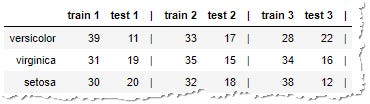The groups are not balanced and sometimes we train on many Setosas while we’re testing on many Versicolors.

The groups are still not balanced. You are often training on a much higher number of samples of one type while testing on different types. Let’s see if we can do something about that.

## Stratified KFold

In many scenarios, it is important to preserve the same distribution of samples in the train and test set. That is achieved by StratifiedKFold which can again be shuffled or unshuffled.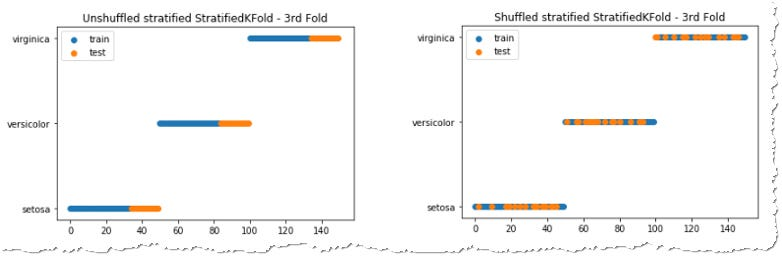Distribution of samples on the third fold of shuffled and unshuffled StratfiedKFold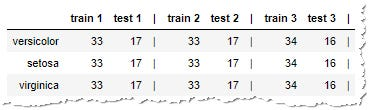Size of train and test set in each fold — Stratification balances the distribution

You can see that the KFold divides the data into the groups which have kept the ratios. StratifiedKFold reflects the distribution of the target variable even in case some of the values appear more often in the dataset. It doesn’t however evaluate the distribution of the input measurements. We will talk more about that at the end.

## Do I need to split my data every time?

To enjoy the benefits of cross-validation you don’t have to split the data manually. Sklearn offers two methods for quick evaluation using cross-validation. `cross_val_score` returns a list of model scores and `cross_validate` also reports training times.

```# cross_validate also allows to specify metrics which you want to see
for i, score in enumerate(cross_validate(model, X, y, cv=3)["test_score"]):
print(f"Accuracy for the fold no. {i} on the test set: {score}")
```

## Other sklearn split options

Besides the functions mentioned above, sklearn is endowed with a whole bunch of other methods to help you address specific needs.

Repeated Kfold would create multiple combinations of train-test split.

While regular cross-validation makes sure that you see each data point once in the test set, ShuffleSplit allows you to specify how many features are picked to test in each fold.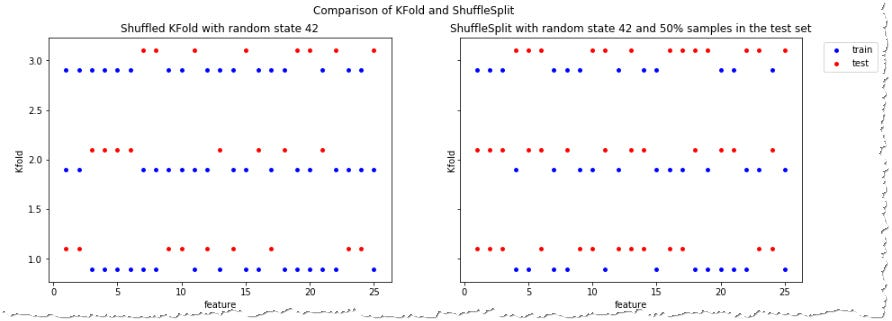While Kfold places each data point into a test set once, using ShuffleSplit you can set % of data going to the test. Here it’s set to 50%.

LeaveOneOut and LeavePOut solve the need in other special cases. The first one always leaves only one sample to be in the test set.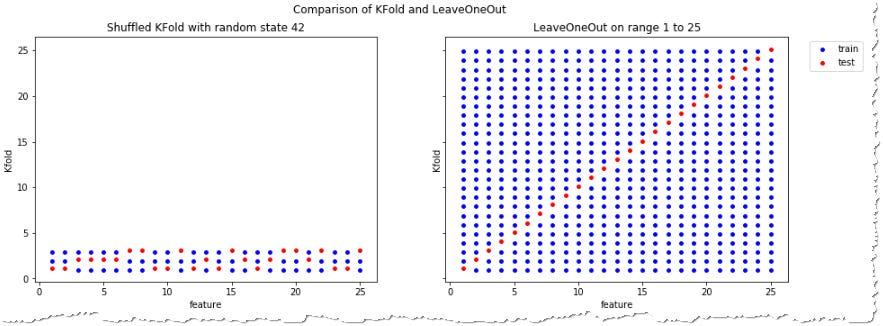LeaveOneOut puts only a single value to test set in each fold.

As a general rule, most authors, and empirical evidence, suggest that 5- or 10- fold cross validation should be preferred to LOO. — sklearn documentation

Group Kfolds

GroupKFold has its place in scenarios when you have multiple data samples taken from the same subject. For example more than one measurement from the same person. It’s likely that data from the same group will behave similarly and if you will train on one of the measurements and test on the other you will get a good score, but it won’t prove that your model generalizes well. GroupKFold assures that the whole group goes either to the train or to the test set. Read more in sklearn documentation about groups.

## Time Series data

Problems involving time series are also sensitive to the order of data points. It’s usually much easier to guess the past based on current knowledge than to predict the future. For this reason, it makes sense to always feed the time series models with older data while predicting the newer ones. Sklearn’s TimeSeriesSplit does exactly that.

## Does stratification consider the input features?

There is one last matter which is crucial to highlight. You might think that the stratified split would solve all your machine learning problems, but it’s not true. StratifiedKFold ensures that there remains the same ratio of the targets in both train and test sets. In our case 33% of each type of Iris.

To demonstrate that on an unbalanced dataset we will look at the popular Kaggle Titanic competition. Your goal would be to train an AI model that would predict whether a passenger on the Titanic survived or died when the ship sank. Let’s look at how StratiffiedKFold would divide the survivors and victims in the dataset in each fold.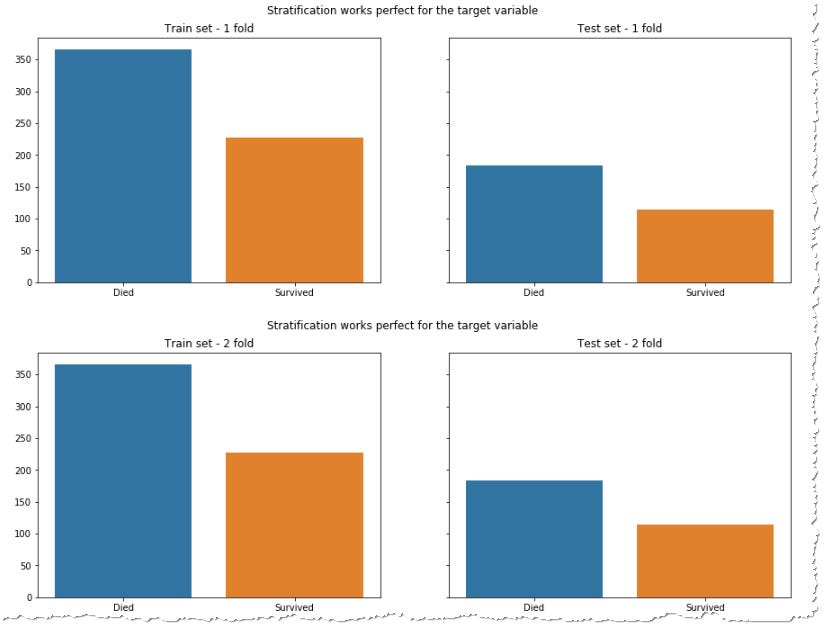The target variable ratio is the same in the train and test set

It looks good, isn’t it? However, your data might still be improperly split. If you look at the distribution of the key features (I purposefully choose this distribution to demonstrate my point, because often it’s enough to shuffle the data to get a much more balanced distribution) you will see that you often try to predict the results based on training data which are different from the test set. For example, if you look at the distribution of the genders in the train and test sets.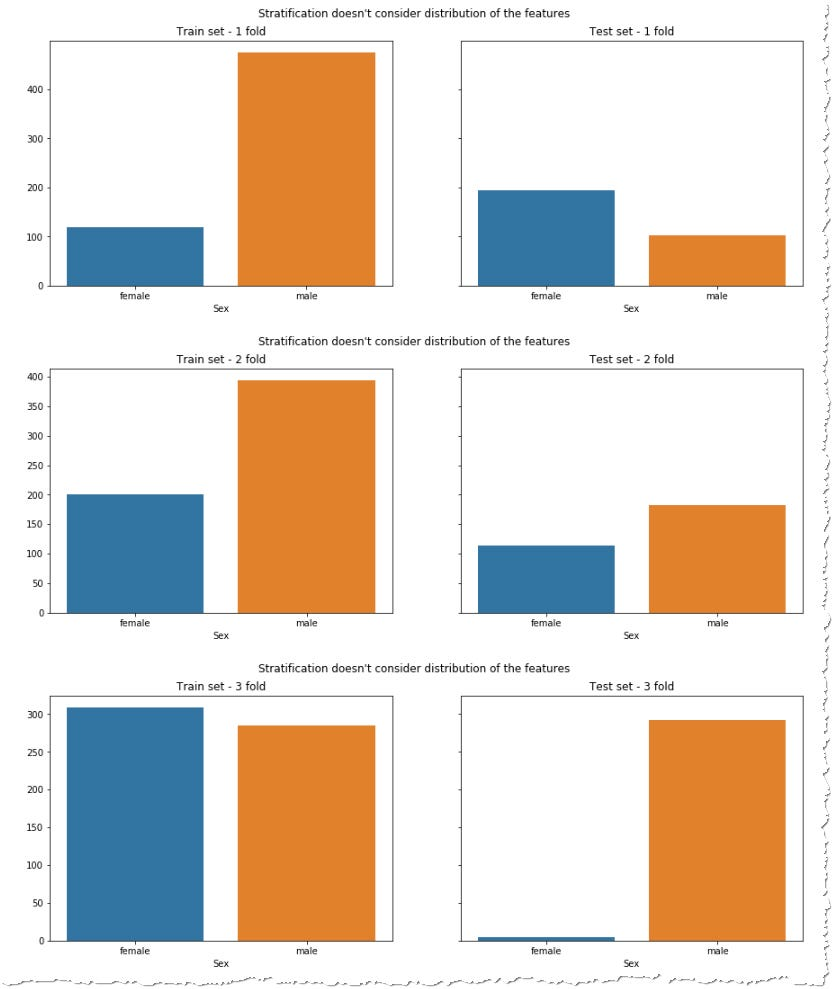Females were more likely to survive the ship’s tragedy. While the first fold learns about a few females and tries to predict the survival of many, the third fold does the opposite.

Cross-validation at least helps you to realize this problem in case the score of the model differs significantly for each fold. Imagine you would be so unlucky, using only a single split that would perfectly suit your test data, but catastrophically fail in real-world scenarios. It is a very complex task to balance your data so that you train and test on ideal distribution. Many argue that it’s not necessary, because the model should generalize well enough to work on the unknown data.

I would nevertheless encourage you to think about the distribution of features. Imagine that you would have a shop where customers are mostly men and you would try to predict sales using data from a marketing campaign targeted to women. It wouldn’t be the best model for your shop.

Conclusion

Train-test split is a basic concept in many machine learning assignments, but if you have enough resources consider applying cross-validation to your problem. It will not only help you use less data, but an inconsistent score on the different folds would suggest that you have missed some important relation inside your data.

Sklearn library contains a bunch of methods to split the data to fit your AI exercise. You can create basic KFold, shuffle the data, or stratify them according to the target variable. You can use additional methods or just test your model with `cross_validate` or `cross_val_score` without bothering with manual data split. In any case, your resulting score should show a stable pattern, because you don’t want your model to depend on ‘lucky’ data split to perform well. All data, charts, and python processing were summarized in the notebook available on Github.

https://towardsdatascience.com/complete-guide-to-pythons-cross-validation-with-examples-a9676b5cac12##### Amir Masoud Sefidian
Machine Learning Engineer